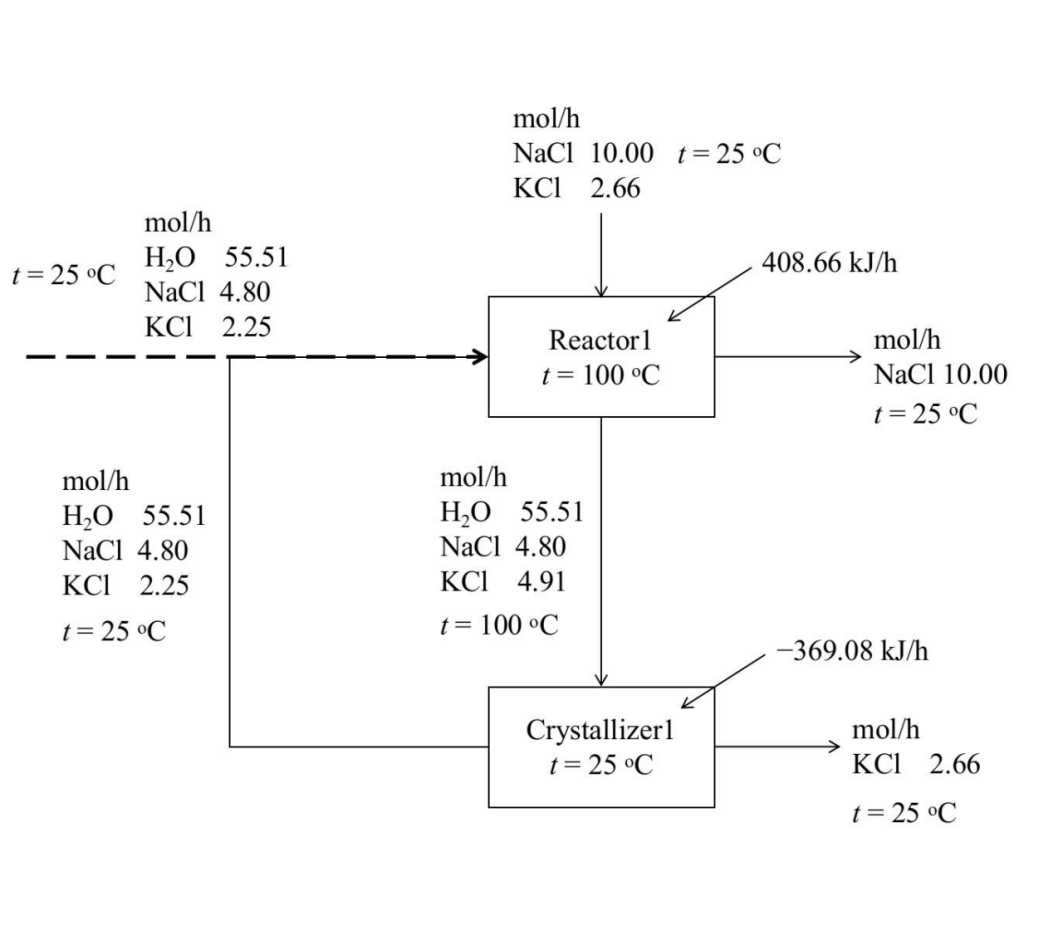# Application(应用案例)

•The left slider shows some applications of the ISLEC software on phase diagram calculation and process simulation. The graphs are not generated directly from the software, but visualized using excel and gnuplot according to the numerical output from ISLEC.

• Process simulation of KCl production from sylvite mineral
• Phase diagram LiCl+NaCl+H2O system
• Phase diagram LiCl+KCl+H2O system
• Phase diagram LiCl+MgCl2+H2O system
• Phase diagram LiCl+CaCl2+H2O system
• Phase diagram NaCl+MgCl2+H2O system
• Phase diagram NaCl+CaCl2+H2O system
• Phase diagram KCl+MgCl2+H2O system
• Phase diagram KCl+CaCl2+H2O system
• Phase diagram MgCl2+CaCl2+H2O system
• Solubility isotherms of H3BO3+NaCl+H2O system
• Solubility isotherms of H3BO3+KCl+H2O system
• Phase diagram of NaOH+H2O system
• Phase diagram of FeSO4+H2O system